# MA.8.NSO.1.1Export Print
Extend previous understanding of rational numbers to define irrational numbers within the real number system. Locate an approximate value of a numerical expression involving irrational numbers on a number line.

### Examples

Within the expression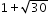, the irrational number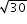can be estimated to be between 5 and 6 because 30 is between 25 and 36. By considering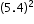and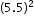, a closer approximation foris 5.5. So, the expressionis equivalent to about 6.5.

### Clarifications

Clarification 1: Instruction includes the use of number line and rational number approximations, and recognizing pi (π) as an irrational number.

Clarification 2: Within this benchmark, the expectation is to approximate numerical expressions involving one arithmetic operation and estimating square roots or pi (π).

General Information
Subject Area: Mathematics (B.E.S.T.)
Strand: Number Sense and Operations
Status: State Board Approved

## Benchmark Instructional Guide

### Terms from the K-12 Glossary

• Expression
• Irrational Numbers
• Number Line
• Pi
• Rational Numbers
• Real Numbers

### Vertical Alignment

Previous Benchmarks

Next Benchmarks

### Purpose and Instructional Strategies

In grade 7, students expressed rational numbers using terminating and repeating decimals. In grade 8, students define irrational numbers and determine their approximate location on a number line. In Algebra 1, students will expand their knowledge of the real number system to understand that complex numbers exist from their work with quadratics.
• Instruction includes using and creating a graphic organizer to show the relationship between the subsets of the real number system.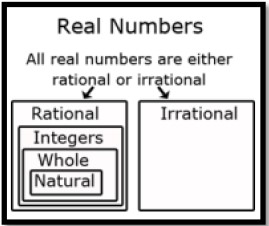• Once students understand that (1) every rational number has a decimal representation that either terminates or repeats, and (2) every terminating or repeating decimal is a rational number, they can reason that on the number line, irrational numbers must have decimal representations that neither terminate nor repeat.
• Students sometimes overgeneralize that all square roots are rational numbers concluding that irrational numbers are unusual and rare. Instruction includes a variety of examples of irrational numbers. Irrational numbers are much more plentiful than rational numbers, in the sense that they are denser in the real number line.
• Instruction includes the understanding that the square root of a whole number is either another whole number or is irrational. When the result is another whole number, the original whole number is a perfect square. This fact is particularly relevant when the Pythagorean Theorem is applied to find a missing side length of a triangle whose other two side lengths are whole numbers.
• Instruction includes the understanding that the cube root of a whole number is either another whole number or is irrational. When the result is another whole number, the original whole number is a perfect cube.
• Instruction includes the understanding that adding or subtracting a rational number and an irrational number produces an irrational number. The same is true of multiplication or division unless the rational number is 0.
• Students should develop estimating skills when working with square roots without the use of a calculator. One strategy is to use benchmark square roots to determine an approximate value.
• For example, to find an approximation of $\sqrt{28}$, first determine the perfect squares 28 is between, which would be 25 and 36. The square roots of 25 and 36 are 5 and 6, respectively, so we know that $\sqrt{28}$ is between 5 and 6. Since 28 is closer to 25, an estimate of the square root would be closer to 5.

### Common Misconceptions or Errors

• Students may incorrectly believe that pi (π) is a rational number since they have only been introduced to a decimal approximation and a fraction approximation. To address this misconception, instruction includes looking further at the decimal representation of pi (π) so that students will notice that a pattern will not emerge. In fact, a pattern never emerges, therefore, pi is irrational.
• Students may incorrectly think that the number line only has the numbers that are labeled.
• Students may incorrectly think a numerical expression that includes addition or subtraction cannot be placed on a number line.
• For example, 2 + $\sqrt{3}$ can be placed on a number line at approximately 3.73.

### Strategies to Support Tiered Instruction

• Teacher provides opportunities to look at the decimal representation of pi (π) and comparing decimal representations of rational numbers and irrational numbers.
• Teachers provide opportunities for practice with performing decimal expansion of rational and irrational numbers to check for a pattern.
• Teacher models how to estimate a numerical expression with addition or subtraction and locates a place on the number line.
• o For example, show a representation of pi (π) and compare it to a decimal representation of $\frac{\text{22}}{\text{7}}$. Students should identify that $\frac{\text{1}}{\text{3}}$ is a rational number and repeats 0.3333, and that $\frac{\text{22}}{\text{7}}$ is pi (π = 3.1415…) and doesn’t repeat.
• For example, a first approximation of 3 + $\sqrt{5}$, students could approximate $\sqrt{5}$ as 2.25 since 5 is between the perfect squares of 4 and 9, but closer to 4. Therefore $\sqrt{5}$ would be in between 2 and 3, but closer to 2. So, a reasonable guess for $\sqrt{5}$ can be 2.25 and therefore a reasonable estimate for 3 + $\sqrt{5}$ would be 3 + 2.25 which equals 5.25. If more accuracy is required, students should understand that a calculator is needed.
• Instruction includes looking further at the decimal representation of pi (π) so that students will notice that a pattern will not emerge. In fact, a pattern never emerges, therefore, pi is irrational.

Part A. Provide an example of a rational number and explain how you can determine that it is rational.
Part B. Choose which number(s) below are irrational and explain how you can determine that they are irrational.
a) $\sqrt{3}$ − 2
b) 6$\sqrt{25}$
c) $\sqrt{36}$
d) 2π
e) −4 + $\sqrt{-216}$

Part A. Use the number lines below to estimate the value of $\sqrt{8}$. Explain why you put the points where you did.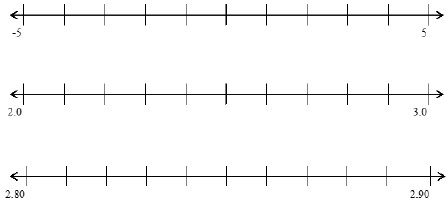Part B. Plot 1 + $\sqrt{8}$ on a number line. Explain your process with a partner.

### Instructional Items

Instructional Item 1
Plot the following numbers on a number line showing their approximate location to the nearest hundredth.
a. π − 2
b. − ($\frac{\text{1}}{\text{2}}$ π)2
c. 2$\sqrt{2}$
d. 2 + $\sqrt{17}$

Instructional Item 2

*The strategies, tasks and items included in the B1G-M are examples and should not be considered comprehensive.

## Related Courses

This benchmark is part of these courses.
1200400: Foundational Skills in Mathematics 9-12 (Specifically in versions: 2014 - 2015, 2015 - 2022, 2022 and beyond (current))
1205050: M/J Accelerated Mathematics Grade 7 (Specifically in versions: 2014 - 2015, 2015 - 2020, 2020 - 2022, 2022 and beyond (current))
1205070: M/J Grade 8 Pre-Algebra (Specifically in versions: 2014 - 2015, 2015 - 2022, 2022 and beyond (current))
1204000: M/J Foundational Skills in Mathematics 6-8 (Specifically in versions: 2014 - 2015, 2015 - 2022, 2022 and beyond (current))
7812030: Access M/J Grade 8 Pre-Algebra (Specifically in versions: 2014 - 2015, 2015 - 2018, 2018 - 2019, 2019 - 2022, 2022 and beyond (current))

## Related Access Points

Alternate version of this benchmark for students with significant cognitive disabilities.
MA.8.NSO.1.AP.1: Locate approximations of irrational numbers on a number line.

## Related Resources

Vetted resources educators can use to teach the concepts and skills in this benchmark.

## Formative Assessments

Sum of Rational Numbers:

Students are asked to define a rational number and then explain why the sum of two rational numbers is rational.

Type: Formative Assessment

Sum of Rational and Irrational Numbers:

Students are asked to describe the difference between rational and irrational numbers and then explain why the sum of a rational and an irrational number is irrational.

Type: Formative Assessment

Product of Non-Zero Rational and Irrational Numbers:

Students are asked to describe the difference between rational and irrational numbers, and then explain why the product of a non-zero rational and an irrational number is irrational.

Type: Formative Assessment

The Root of the Problem:

Students are asked to evaluate perfect square roots and perfect cube roots.

Type: Formative Assessment

Rational Numbers:

Students are asked to identify rational numbers from a list of real numbers, explain how to identify rational numbers, and to identify the number system that contains numbers that are not rational.

Type: Formative Assessment

Decimal to Fraction Conversion:

Students are given several terminating and repeating decimals and asked to convert them to fractions.

Type: Formative Assessment

## MFAS Formative Assessments

Decimal to Fraction Conversion:

Students are given several terminating and repeating decimals and asked to convert them to fractions.

Product of Non-Zero Rational and Irrational Numbers:

Students are asked to describe the difference between rational and irrational numbers, and then explain why the product of a non-zero rational and an irrational number is irrational.

Rational Numbers:

Students are asked to identify rational numbers from a list of real numbers, explain how to identify rational numbers, and to identify the number system that contains numbers that are not rational.

Sum of Rational and Irrational Numbers:

Students are asked to describe the difference between rational and irrational numbers and then explain why the sum of a rational and an irrational number is irrational.

Sum of Rational Numbers:

Students are asked to define a rational number and then explain why the sum of two rational numbers is rational.

The Root of the Problem:

Students are asked to evaluate perfect square roots and perfect cube roots.

## Student Resources

Vetted resources students can use to learn the concepts and skills in this benchmark.

## Parent Resources

Vetted resources caregivers can use to help students learn the concepts and skills in this benchmark.